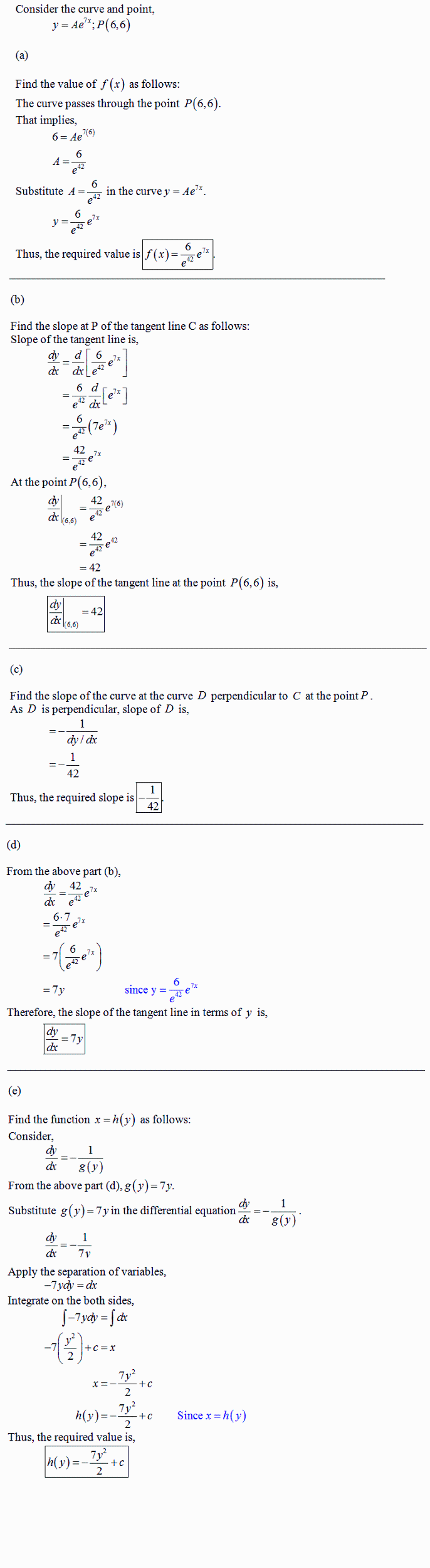Question# Consider the curves in the first quadrant that have equationsy=Aexp(7x), where A is a positive constant. Different valuesof A give different curves. T

Other
ANSWEREDConsider the curves in the first quadrant that have equationsy=Aexp(7x), where A is a positive constant. Different valuesof A give different curves. The curves form a family,F. Let P=(6,6). Let C be the number of the family Fthat goes through P.
A. Let y=f(x) be the equation of C. Find f(x).
B. Find the slope at P of the tangent to C.
C. A curve D is a perpendicular to C at P. What is the slope of thetangent to D at the point P?
D. Give a formula g(y) for the slope at (x,y) of the member of Fthat goes through (x,y). The formula should not involve A orx.
E. A curve which at each of its points is perpendicular to themember of the family F that goes through that point is called anorthogonal trajectory of F. Each orthogonal trajectory to Fsatisfies the differential equation dy/dx = -1/g(y), where g(y) isthe answer to part D.
Find a function of h(y) such that x=h(y) is the equation of theorthogonal trajectory to F that passes through the point P.2021-05-182021-09-08

Consider the curve and point,

$$y=Ae^{7x};P(6,6)$$

a) Find the value of f(x) as follows:

The curve passes through the point P(6,6).

That implies,

$$6=Ae^{7(6)}$$

$$A=\frac{6}{e^{42}}$$

Substitute $$A=\frac{6}{e^{42}}$$ in the curve $$y=Ae^{7x}$$.

$$y=\frac{6}{e^{42}}e^{7x}$$

Thus, the required value is $$f(x)=\frac{6}{e^{42}}e^{7x}$$

b) Find the slope at P of tangent line C as follows:

Slope of the tangent line is,

$$\frac{dy}{dx}=\frac{d}{dx}[\frac{6}{e^{42}}e^{7x}]$$

$$=\frac{6}{e^{42}}\frac{d}{dx}[e^{7x}]$$

$$=\frac{6}{e^{42}}(7e^{7x})$$

$$=\frac{42}{e^{42}}e^{7x}$$

At the point $$P(6,6),$$

$$\frac{dy}{dx}|_{(6,6)}=\frac{42}{e^{42}}e^{7(6)}$$

$$=\frac{42}{e^{42}}e^{42}$$

$$=42$$

Thus, the slope of the tangent line at the point $$P(6,6)$$ is,

$$\frac{dy}{dx}|_{(6,6)}=42$$

c) Find the slope of the curve at the curve D perpendicular to C at the point P.

As D is perpendicular, slope of D is,

$$=\frac{1}{dy/dx}$$

$$=-\frac{1}{42}$$

Thus, the required slope is $$-\frac{1}{42}$$

d) From the above part (b),

$$\frac{dy}{dx}=\frac{42}{e^{42}}e^{7x}$$

$$=\frac{6\cdot7}{e^{42}}e^{7x}$$

$$=7(\frac{6}{e^{42}}e^{7x})$$

$$=7y$$

Therefore, the slope of the tangent line in terms of y is,

$$\frac{dy}{dx}=7y$$

e) Find the function $$x=h(y)$$ as follows:

Consider,

$$\frac{dy}{dx}=-\frac{1}{g(y)}$$

From the above part $$(d),g(y)=7y$$

Substitute $$g(y)=7y$$ in the differential equation $$\frac{dy}{dx}=-\frac{1}{g(y)}$$

$$\frac{dy}{dx}=-\frac{1}{7y}$$

Apply the separation of variables,

$$-7ydy=dx$$

Integrate on the both sides,

$$\int-7ydy=\int dx$$

$$-7(\frac{y^2}{2})+c=x$$

$$x=-\frac{7y^2}{2}+c$$

$$h(y)=-\frac{7y^2}{2}+c$$

Thus, the required values is,

$$h(y)=-\frac{7y^2}{2}+c$$